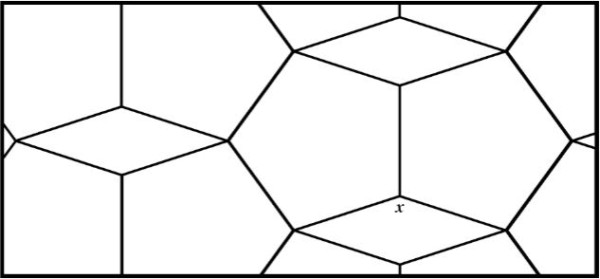# Word Problem Wednesday: All In Good Measure

By Marketing | Added Nov 4, 2020Measuring allows us to find out the extent, quantity, amount, or degree of something. And we use our abilities to measure in a lot of different ways. From figuring out what size of clothes we should wear — to buying the right sized couch for our homes, we rely on measurement to make things fit.

This week’s word problems will put your child’s ability to measure to the test. Read the problems below and choose the one that’s the right skill level for your child. Have them give it a try. And when they feel they’ve found the answer, check their solution against ours.

# Questions

Lower Elementary:
Question: Tyler has a sticker book that is 8 inches wide and 9 inches tall. He has a set of stickers that are each 2 inches wide and 3 inches tall.  How many stickers will fit on a page of the sticker book?

Upper Elementary:
Question: Alli has a box that is exactly the right size to hold 7 layers of 9 rows of 11 cube-shaped erasers. How many erasers fit inside the box?

Middle School:
Question: Bobby is riding his tricycle around a quarter-mile track. If each wheel is 12 inches in diameter and turns 100 times, then how much further does Bobby need to pedal in order to complete a lap around the track?

Algebra and Up:
Question: The tessellating pattern below comprises regular pentagons and rhombuses. Find the measure of x.# Solutions

Excellent!  Are you ready to check your child’s answer? Look below to see if their solution matches ours.

Lower Elementary:

Solution:  Since each sticker is 2 inches wide and each page is 8 inches wide, that means Tyler can fit 8 ÷ 2 = 4 stickers in each row. Since the page is 9 inches tall and each sticker is 3 inches tall, that means Tyler can fit 9 ÷ 3 = 3 rows of stickers on each page. So, Tyler can fit 4, 8, 12 stickers on each page of his sticker book.

Upper Elementary:
Solution:  The length of the box is equal to 11 erasers, the width is equal to 9 erasers, and the depth is equal to 7 erasers. So, the box will hold 11 × 9 × 7 = 693 erasers in total.

Middle School:
Solution:  Each time the wheels rotate, Bobby moves forward 2πr ≈ 2 × 3.14 × ½ foot = 3.14 feet. Since a mile is 5,280 feet, the track is 5,280 ÷ 4 = 1,320 feet. If Bobby turns his wheels 100 times, he will travel 314 feet, which means he will have 1,320 – 314 = 1,006 feet left to complete a lap around the track.

Algebra and Up:

Solution:  Since the sum of the measures of all 5 vertices of a pentagon is 540°, that means that each angle is 540° ÷ 5 = 108°. Two 108° angles and x make a full 360° circle, so 360° – 108° × 2 = 360° – 216° = 144°. So, the measure of x is 144°.